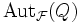# Radical subgroup for a fusion system

Suppose$P$ is a group of prime power order and$\mathcal{F}$ is a fusion system on$P$. A subgroup$Q$ of$P$ is termed a$\mathcal{F}$-radical subgroup if$O_p(\operatorname{Aut}_{\mathcal{F}}(Q)) = \operatorname{Aut}_Q(Q)$. Here,$\operatorname{Aut}_{\mathcal{F}}(Q)$ denotes the automorphisms of$Q$ in the category$\mathcal{F}$,$O_p$ denotes the p-core, and$\operatorname{Aut}_Q(Q)$ denotes the inner automorphism group of$Q$, viewed as a subgroup of$\operatorname{Aut}_{\mathcal{F}}(Q)$.